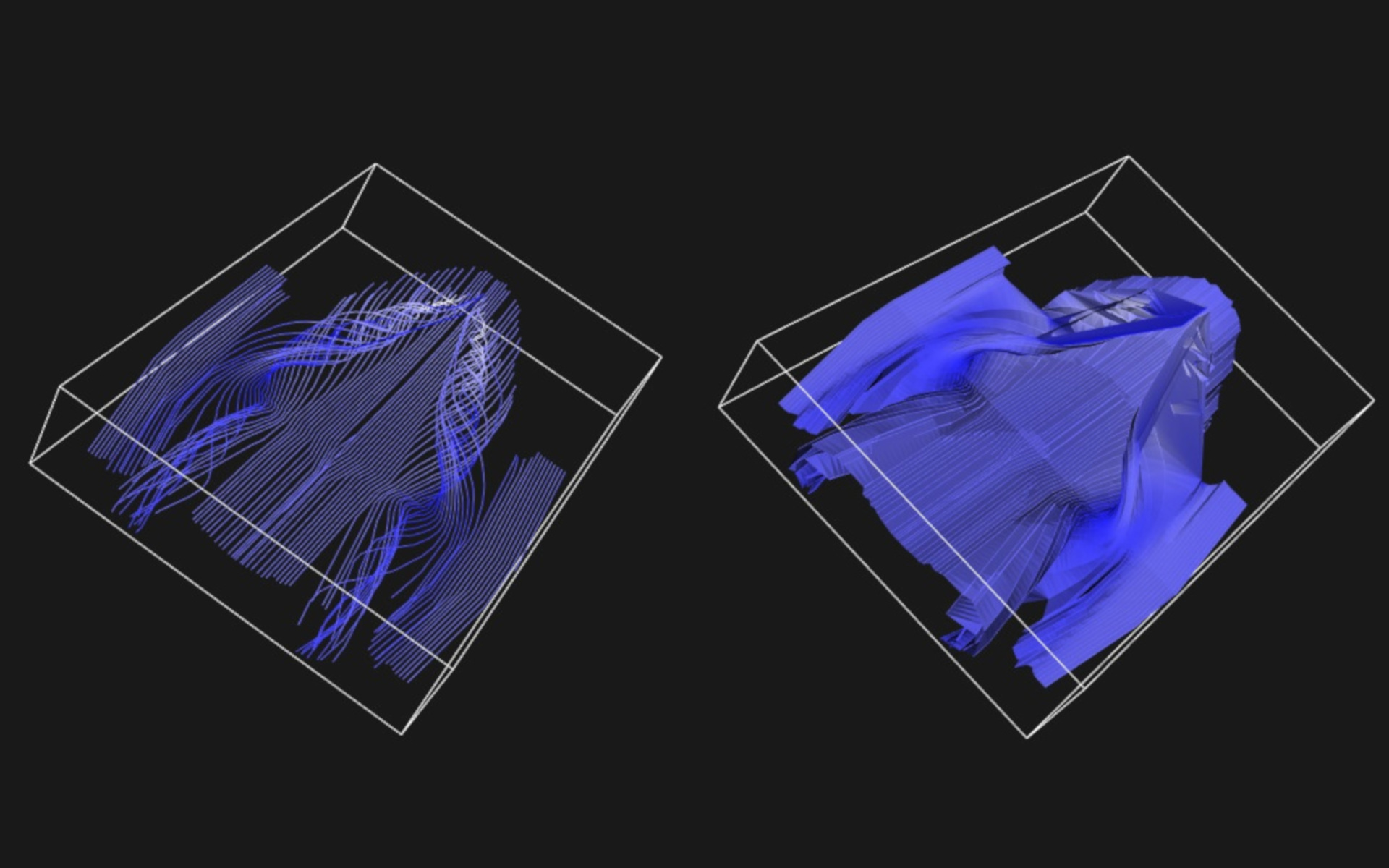3D Flow Visualization
Date04/10/2017
Author(s)Andres Bejarano

A 3D flow visualization tool based on Runge-Kutta 4 (RK4) and Greedy Tiling for Streamsurface reconstruction. Developed using C++ and VTK.

Instructions: After executing the program a console window will open asking for different parameters for visualizing the vector field:

• - The step size h for the Runge Kutta 4 (RK4) algorithm. Values between 0.05 and 1 are recommended for a good level of detail in the streamlines.
• - The number of seeds (points) to be uniformly placed along the line segment for generating the streamlines. Values between 70 and 200 are recommended for a good level of detail of the vector field flow.
• - Generating the stream surface. Enter 0 if only the streamlines are required; otherwise, enter 1 for displaying the stream surface.

The system reads the vtk file and displays the physical origin point of the vector field and its physical dimensions. For the delta.vtk file such values are (-100, -200, 0) and (502.007, 202.01, 101.01) respectively. These points are also the end points of a diagonal line segment traversing the vector field. The system will ask if such diagonal is good for generating the seeds. Enter 0 for No or 1 for Yes. In case that No was the selected option the system will ask for the start and end points of the line segment. Any two points within the bounding box of the vector field will work. When entering the points separate the coordinate values using space only (i.e., 300 -200 50).
For visualizing the vector field the program generates the specified amount of seeds (points) uniformly along the line segment between the indicated start and end points. From each one of the seeds it is calculated the streamlines in both positive and negative directions until the points in the streamline go outside of the bounding box. The speed of the vector field is visualized using different colors: white for high speed and blue for low speed. Such speed values are determined by the magnitude of the vector field in each one of the points in the streamlines. The respective vector values for the streamline points are calculated using trilinear interpolation. The flow of the streamlines is calculated using the RK4 algorithm using the specified h value.

Stream surface generation is performed using the Greedy Tiling approach described in Hultquist 1992. For two adjacent streamlines, a ribbon made of triangles is generated. Such triangles must comply with a restriction of minimal diagonal length between both streamlines. On each iteration of the algorithm it is kept two consecutive points for each streamline: L0 and L1 for the left streamline, and R0 and R1 for the right streamline. Such four points form a quadrilateral joining the streamlines. Since the quadrilateral must be split for generating the triangle then the algorithm must select which diagonal (L0R1 or L1R0) to use. The shortest of the two diagonals is selected for forming the required triangle. The process continues until the end points in both streamlines are reached. The colors of the stream surface are based on the colors for the points in the streamlines.